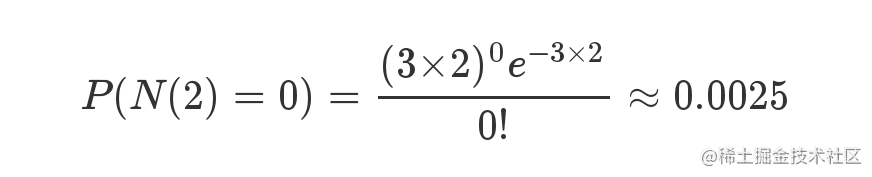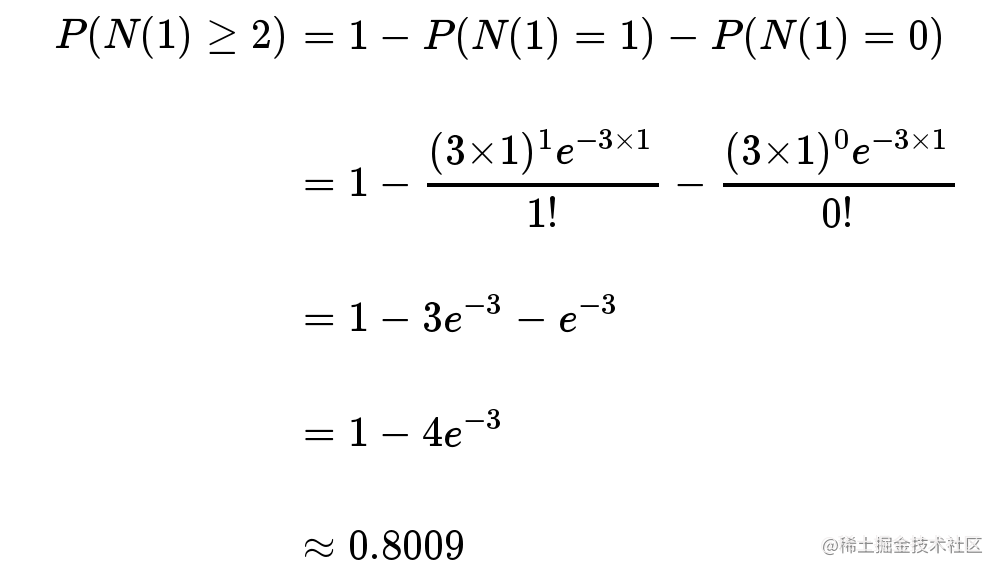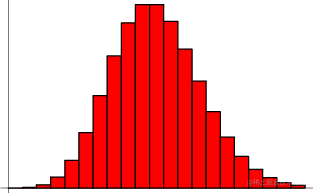# 用泊松分布来解释为什么HashMap的链表变树的阈值是8

## 前言## 泊松分布## HashMap的链表变树阈值为什么是8

HashMap的key碰撞问题，每次key的碰撞，都可以认为是一次独立事件。与上次或下次是否发生key碰撞，都无关系。• e^-0.5 = 0.6065

$P(N(1)=k) = 0.6065*(0.5^k)/k!$

• 当k=0时，P= 0.6065
• 当k=1时，p= 0.3032
• 当k=2时，p= 0.0758
• 当k=3时，p= 0.0126
• 当k=4时，p= 0.0015
• 当k=5时，p= 0.0001
• 当k=6时，p= 0.000013
• 当k=7时，p= 0.0000009
• 当k=8时，p= 0.00000006
• 当k=9时，p= 0.000000003

    * 0:    0.60653066
* 1:    0.30326533
* 2:    0.07581633
* 3:    0.01263606
* 4:    0.00157952
* 5:    0.00015795
* 6:    0.00001316
* 7:    0.00000094
* 8:    0.00000006
* more: less than 1 in ten million

## 参考资料

• 维基百科
• 阮一峰的网络日志——《泊松分布和指数分布：10分钟教程》